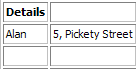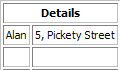# QTextTable Class

The QTextTable class represents a table in a QTextDocument. More...

 Header: #include qmake: QT += gui Inherits: QTextFrame

Note: All functions in this class are reentrant.

## Public Functions

 void appendColumns(int count) void appendRows(int count) QTextTableCell cellAt(int row, int column) const QTextTableCell cellAt(int position) const QTextTableCell cellAt(const QTextCursor &cursor) const int columns() const QTextTableFormat format() const void insertColumns(int index, int columns) void insertRows(int index, int rows) void mergeCells(int row, int column, int numRows, int numCols) void mergeCells(const QTextCursor &cursor) void removeColumns(int index, int columns) void removeRows(int index, int rows) void resize(int rows, int columns) QTextCursor rowEnd(const QTextCursor &cursor) const QTextCursor rowStart(const QTextCursor &cursor) const int rows() const void setFormat(const QTextTableFormat &format) void splitCell(int row, int column, int numRows, int numCols)

## Detailed Description

A table is a group of cells ordered into rows and columns. Each table contains at least one row and one column. Each cell contains a block, and is surrounded by a frame.

Tables are usually created and inserted into a document with the QTextCursor::insertTable() function. For example, we can insert a table with three rows and two columns at the current cursor position in an editor using the following lines of code:

```    QTextCursor cursor(editor->textCursor());
cursor.movePosition(QTextCursor::Start);

QTextTable *table = cursor.insertTable(rows, columns, tableFormat);```

The table format is either defined when the table is created or changed later with setFormat().

The table currently being edited by the cursor is found with QTextCursor::currentTable(). This allows its format or dimensions to be changed after it has been inserted into a document.

A table's size can be changed with resize(), or by using insertRows(), insertColumns(), removeRows(), or removeColumns(). Use cellAt() to retrieve table cells.

The starting and ending positions of table rows can be found by moving a cursor within a table, and using the rowStart() and rowEnd() functions to obtain cursors at the start and end of each row.

Rows and columns within a QTextTable can be merged and split using the mergeCells() and splitCell() functions. However, only cells that span multiple rows or columns can be split. (Merging or splitting does not increase or decrease the number of rows and columns.)

Note that if you have merged multiple columns and rows into one cell, you will not be able to split the merged cell into new cells spanning over more than one row or column. To be able to split cells spanning over several rows and columns you need to do this over several iterations.Suppose we have a 2x3 table of names and addresses. To merge both columns in the first row we invoke mergeCells() with row = 0, column = 0, numRows = 1 and numColumns = 2.` table->mergeCells(0, 0, 1, 2);`This gives us the following table. To split the first row of the table back into two cells, we invoke the splitCell() function with numRows and numCols = 1.` table->splitCell(0, 0, 1, 1);`This results in the original table.

## Member Function Documentation

### void QTextTable::appendColumns(intcount)

Appends count columns at the right side of the table.

This function was introduced in Qt 4.5.

### void QTextTable::appendRows(intcount)

Appends count rows at the bottom of the table.

This function was introduced in Qt 4.5.

### QTextTableCell QTextTable::cellAt(introw, intcolumn) const

Returns the table cell at the given row and column in the table.

### QTextTableCell QTextTable::cellAt(intposition) const

Returns the table cell that contains the character at the given position in the document.

### QTextTableCell QTextTable::cellAt(const QTextCursor &cursor) const

Returns the table cell containing the given cursor.

### int QTextTable::columns() const

Returns the number of columns in the table.

### QTextTableFormat QTextTable::format() const

Returns the table's format.

### void QTextTable::insertColumns(intindex, intcolumns)

Inserts a number of columns before the column with the specified index.

### void QTextTable::insertRows(intindex, introws)

Inserts a number of rows before the row with the specified index.

### void QTextTable::mergeCells(introw, intcolumn, intnumRows, intnumCols)

Merges the cell at the specified row and column with the adjacent cells into one cell. The new cell will span numRows rows and numCols columns. This method does nothing if numRows or numCols is less than the current number of rows or columns spanned by the cell.

This function was introduced in Qt 4.1.

### void QTextTable::mergeCells(const QTextCursor &cursor)

Merges the cells selected by the provided cursor.

This function was introduced in Qt 4.1.

### void QTextTable::removeColumns(intindex, intcolumns)

Removes a number of columns starting with the column at the specified index.

### void QTextTable::removeRows(intindex, introws)

Removes a number of rows starting with the row at the specified index.

### void QTextTable::resize(introws, intcolumns)

Resizes the table to contain the required number of rows and columns.

### QTextCursor QTextTable::rowEnd(const QTextCursor &cursor) const

Returns a cursor pointing to the end of the row that contains the given cursor.

### QTextCursor QTextTable::rowStart(const QTextCursor &cursor) const

Returns a cursor pointing to the start of the row that contains the given cursor.

### int QTextTable::rows() const

Returns the number of rows in the table.

### void QTextTable::setFormat(const QTextTableFormat &format)

Sets the table's format.